Processing ......FreeComputerBooks.com Links to Free Computer, Mathematics, Technical Books all over the World

Discrete Structures for Computer Science: Counting, Recursion, and Probability
🌠 Top Free C Programming Books - 100% Free or Open Source
• Title: Discrete Structures for Computer Science: Counting, Recursion, and Probability
• Author(s) Michiel Smid
• Publisher: Carleton University (Creative Commons Licensed. July 22, 2019)
• Hardcover/Paperback: N/A
• eBook: PDF (404 pages)
• Language: English
• ISBN-10/ASIN: N/A
• ISBN-13: N/A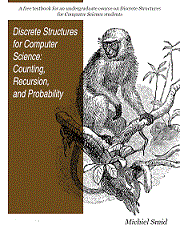Book Description

This book provides a broad introduction to some of the most fascinating and beautiful areas of discrete mathematical structures. It starts with a chapter on sets and goes on to provide examples in logic, applications of the principle of inclusion and exclusion and finally the pigeonhole principal.

Computational techniques including the principle of mathematical introduction are provided, as well as a study on elementary properties of graphs, trees and lattices. Some basic results on groups, rings, fields and vector spaces are also given, the treatment of which is intentionally simple since such results are fundamental as a foundation for students of discrete mathematics. In addition, some results on solutions of systems of linear equations are discussed.

The author strives to change the way logic and discrete math are taught in computer science and mathematics: while many books treat logic simply as another topic of study, this one is unique in its willingness to go one step further. The book traets logic as a basic tool which may be applied in essentially every other area.

• Michiel Smid is a Professor of Computer Science, School of Computer Science, Carleton University, Ottawa, Canada.
Reviews, Ratings, and Recommendations: Related Book Categories: Read and Download Links:Similar Books:
•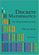Discrete Mathematics: An Open Introduction (Oscar Levin)

This is a gentle introduction to discrete mathematics. Four main topics are covered: counting, sequences, logic, and graph theory. Along the way proofs are introduced, including proofs by contradiction, proofs by induction, and combinatorial proofs.

•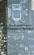Applied Discrete Structures, Fundamentals (Al Doerr, et al)

This book contains most of the fundamental concepts taught in a one semester course in discrete mathematics which is a required course for students in Computer Science, Mathematics and Information Technology.

•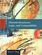Discrete Structures, Logic, and Computability (James L. Hein)

This book contains programming experiments that are designed to reinforce the learning of discrete mathematics, logic, and computability, provides introductions to those ideas and techniques to understand and practice the art and science of computing.

•A Spiral Workbook for Discrete Mathematics (Harris Kwong)

This is a book on discrete mathematics with an emphasis on motivation. It explains and clarifies the unwritten conventions in mathematics, and guides the students through a detailed discussion on how a proof is revised from its draft to a final polished form.

•Mathematics for Computer Science (Eric Lehman, et al)

This book covers elementary discrete mathematics for computer science and engineering. It emphasizes mathematical definitions and proofs as well as applicable methods. explores the topics of basic combinatorics, number and graph theory, logic and proof techniques.

•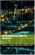Mathematics for Computer Scientists (Gareth J. Janacek, et al)

The aim of this book is to present some the basic mathematics that is needed by computer scientists. The reader is not expected to be a mathematician and we hope will find what follows useful.

•Lists, Decisions and Graphs - With an Introduction to Probability

In this book, four basic areas of discrete mathematics are presented: Counting and Listing (Unit CL), Functions (Unit Fn), Decision Trees and Recursion (Unit DT), and Basic Concepts in Graph Theory (Unit GT).

•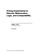Prolog Experiments in Discrete Math, Logic, and Computability

This book contains programming experiments that are designed to reinforce the learning of discrete mathematics, logic, and computability. The Prolog programming language is the tool used for the experiments in this book.

•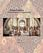Isomorphism - Mathematics of Programming (Xinyu Liu)

This book introduces the mathematics behind computer programming. It intents to tell: programming is isomorphic to mathematics. Just like in art and music, there are interesting stories and mathematicians behind the great minds.

Book Categories
 :All CategoriesTop Free BooksRecent BooksMiscellaneous BooksComputer EngineeringComputer LanguagesComputer ScienceData Science/DatabasesJava and Java EE (J2EE)Linux and UnixMathematicsMicrosoft and .NETMobile ComputingNetworking and CommunicationsSoftware EngineeringSpecial TopicsWeb Programming
Other Categories